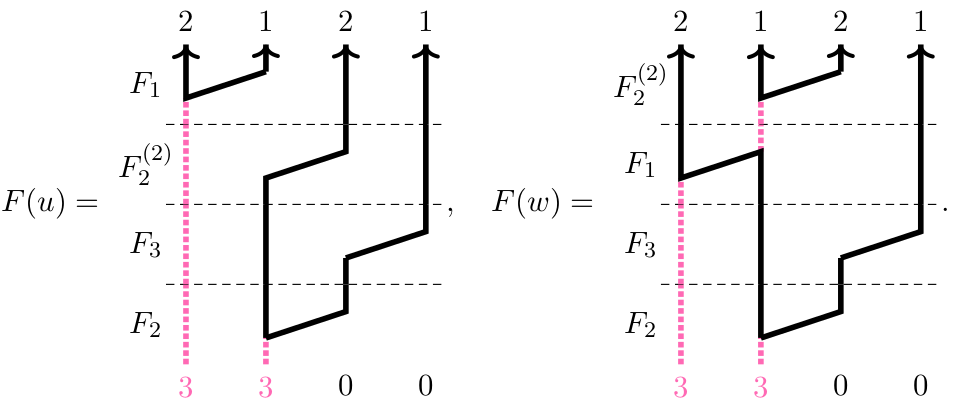## Data

• Title: A formula to evaluate type A webs and link polynomials
• Authors: Abel Lacabanne, Daniel Tubbenhauer and Pedro Vaz
• Status: Preprint. Last update: Sun, 25 Sep 2022 03:24:17 EST
• Source: click
• GitHub for the code: click

## Abstract

We give a closed formula to evaluate exterior webs (also called MOY webs) and the associated Reshetikhin-Turaev link polynomials.

## A few extra words

This work in a combination of https://arxiv.org/abs/1404.5752 and https://arxiv.org/abs/2108.05508.
The Reshetikhin-Turaev invariants of links are one of the most important family of link invariants in quantum topology and its ramifications.
In this note we focus on the subfamily of these invariants given by coloring the strands of links with exterior powers of the vector representation of quantum $$\mathfrak{sl}_n$$ or quantum $$\mathfrak{gl}_n$$. We show that this family of polynomials can be computed by a \emph{closed formula} that takes as input only combinatorial data associated to a fixed colored link diagram and root theoretic data associated to the type A Dynkin diagram. The formula works for all links, all coloring, and all ranks.
Let us stress that, by its very nature, the closed formula we give is a Weyl-character-type formula: On the one hand, it is general, completely algorithmic and reveals abstract properties of the family of exterior colored link polynomials. But on the other hand, the formula does not give an efficient way to compute these invariants, at least not without massaging it a bit.
The diagrammatic incarnation of this family of link invariants is given by (exterior $$\mathfrak{sl}_n$$ or $$\mathfrak{gl}_n$$) webs as shown in many works. In fact, the aforementioned formula is an application of a closed evaluation formula for webs that we will also state and prove. This formula does not need any combinatorics on webs or tableaux; it in fact does not even need the webs at all. Moreover, another application of this formula is an easy to check criterion for webs to represent dual canonical basis elements.
Here is an explicit example. First, bring the webs for which you want to calculate the evaluation into F-form (the choices how to do this does not affect the result):Then the pairing of these two webs is given by the closed formula we state with the input being only the number of columns, the bottom sequence and the F-sequences. There is al SAGEMath code available in the source file to do calculations.